# 6. Continuous probability distributions#

This open-access textbook is, and will remain, freely available for everyone’s enjoyment (also in PDF; a paper copy can also be ordered). It is a non-profit project. Although available online, it is a whole course, and should be read from the beginning to the end. Refer to the Preface for general introductory remarks. Any bug/typo reports/fixes are appreciated. Make sure to check out Deep R Programming  too.

Each successful data analyst will deal with hundreds or thousands of datasets in their lifetime. In the long run, at some level, most of them will be deemed boring. This is because only a few common patterns will be occurring over and over again.

In particular, the previously mentioned bell-shapedness and right-skewness are quite prevalent in the so-called real world. Surprisingly, however, this is exactly when things become scientific and interesting, allowing us to study various phenomena at an appropriate level of generality.

Mathematically, such idealised patterns in the histogram shapes can be formalised using the notion of a probability density function (PDF) of a continuous, real-valued random variable.

Intuitively, a PDF is a smooth curve that would arise if we drew a histogram for the entire population (e.g., all women living currently on Earth and beyond or otherwise an extremely large data sample obtained by independently querying the same underlying data generating process) in such a way that the total area of all the bars is equal to 1 and the bin sizes are very small.

As stated at the beginning, we do not intend this to be a course in probability theory and mathematical statistics. Rather, it precedes and motivates them (e.g., [21, 38, 40, 79]). Therefore, our definitions are out of necessity simplified so that they are digestible. For the purpose of our illustrations, we will consider the following characterisation.

Important

(*) We call an integrable function $$f:\mathbb{R}\to\mathbb{R}$$ a probability density function if $$f(x)\ge 0$$ for all $$x$$ and $$\int_{-\infty}^\infty f(x)\,dx=1$$, i.e., it is nonnegative and normalised in such a way that the total area under the whole curve is 1.

For any $$a < b$$, we treat the area under the fragment of the $$f(x)$$ curve for $$x$$ between $$a$$ and $$b$$, i.e., $$\int_a^b f(x)\,dx$$, as the probability of the underlying real-valued random variable’s (theoretical data generating process’) falling into the $$[a, b]$$ interval.

Some distributions appear more frequently than others and appear to fit empirical data or parts thereof particularly well; compare . In this chapter, we review a few noteworthy probability distributions: the normal, log-normal, Pareto, and uniform families (we will also mention the chi-squared, Kolmogorov, and exponential ones in this course).

## 6.1. Normal distribution#

A normal (Gaussian) distribution has a prototypical, nicely symmetric, bell-shaped density. It is described by two parameters: $$\mu\in\mathbb{R}$$ (the expected value, at which the PDF is centred) and $$\sigma>0$$ (the standard deviation, saying how much the distribution is dispersed around $$\mu$$); compare Figure 6.1.

The probability density function of $$\mathrm{N}(\mu, \sigma)$$ is given by:

$f(x) = \frac{1}{\sqrt{2\pi \sigma^2}} \exp\left( -\frac{(x-\mu)^2}{2\sigma^2} \right).$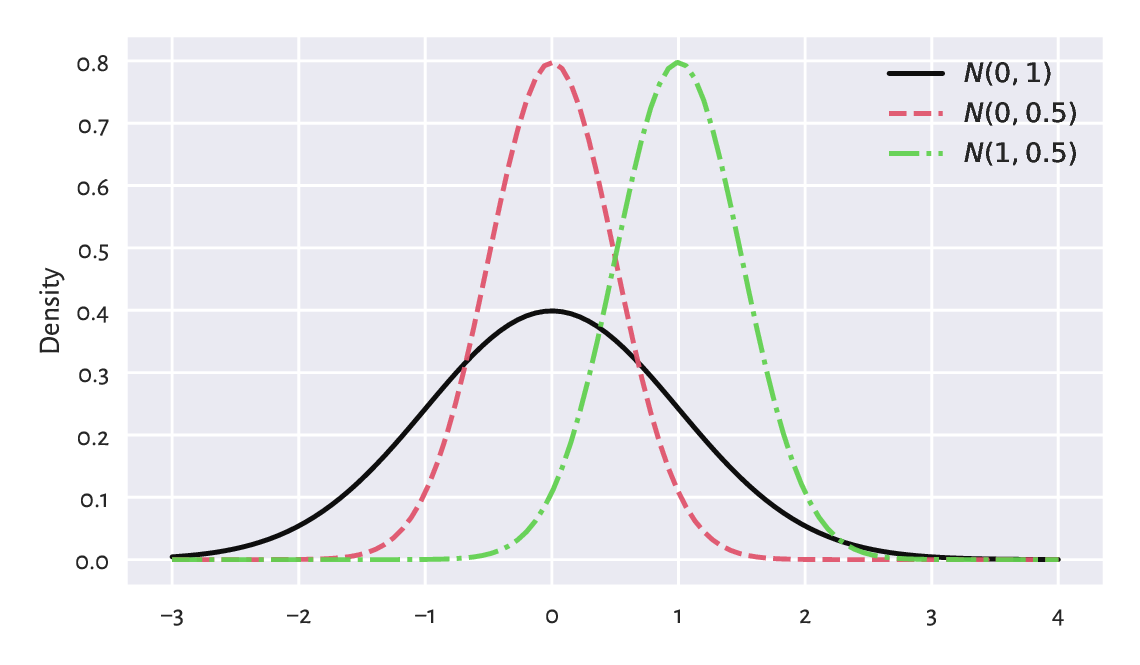Figure 6.1 The probability density functions of some normal distributions $$\mathrm{N}(\mu, \sigma)$$. Note that $$\mu$$ is responsible for shifting and $$\sigma$$ affects scaling/stretching of the probability mass.#

### 6.1.1. Estimating parameters#

A course in statistics (which, again, this one is not, we are merely making an illustration here), may tell us that the sample arithmetic mean $$\bar{x}$$ and standard deviation $$s$$ are natural, statistically well-behaving estimators of the said parameters: if all observations would really be drawn independently from $$\mathrm{N}(\mu, \sigma)$$ each, then we expect $$\bar{x}$$ and $$s$$ to be equal to, more or less, $$\mu$$ and $$\sigma$$ (the larger the sample size, the smaller the error).

Recall the heights (females from the NHANES study) dataset and its bell-shaped histogram in Figure 4.2.

heights = np.genfromtxt("https://raw.githubusercontent.com/gagolews/" +
n = len(heights)
n
## 4221


Let us estimate the said parameters for this sample:

mu = np.mean(heights)
sigma = np.std(heights, ddof=1)
mu, sigma
## (160.13679222932953, 7.062858532891359)


Mathematically, we will denote these two by $$\hat{\mu}$$ and $$\hat{\sigma}$$ (mu and sigma with a hat) to emphasise that they are merely guesstimates of the unknown respective parameters $$\mu$$ and $$\sigma$$. On a side note, in this context, the requested ddof=1 estimator has slightly better statistical properties.

Let us draw the fitted density function (i.e., the PDF of $$\mathrm{N}(160.1, 7.06)$$ which we can compute using scipy.stats.norm.pdf), on top of the histogram; see Figure 6.2. We pass density=True to matplotlib.pyplot.hist to normalise the bars’ heights so that their total area sums to 1.

plt.hist(heights, density=True, color="lightgray", edgecolor="black")
x = np.linspace(np.min(heights), np.max(heights), 1000)
plt.plot(x, scipy.stats.norm.pdf(x, mu, sigma), "r--",
label=f"PDF of N({mu:.1f}, {sigma:.2f})")
plt.ylabel("Density")
plt.legend()
plt.show()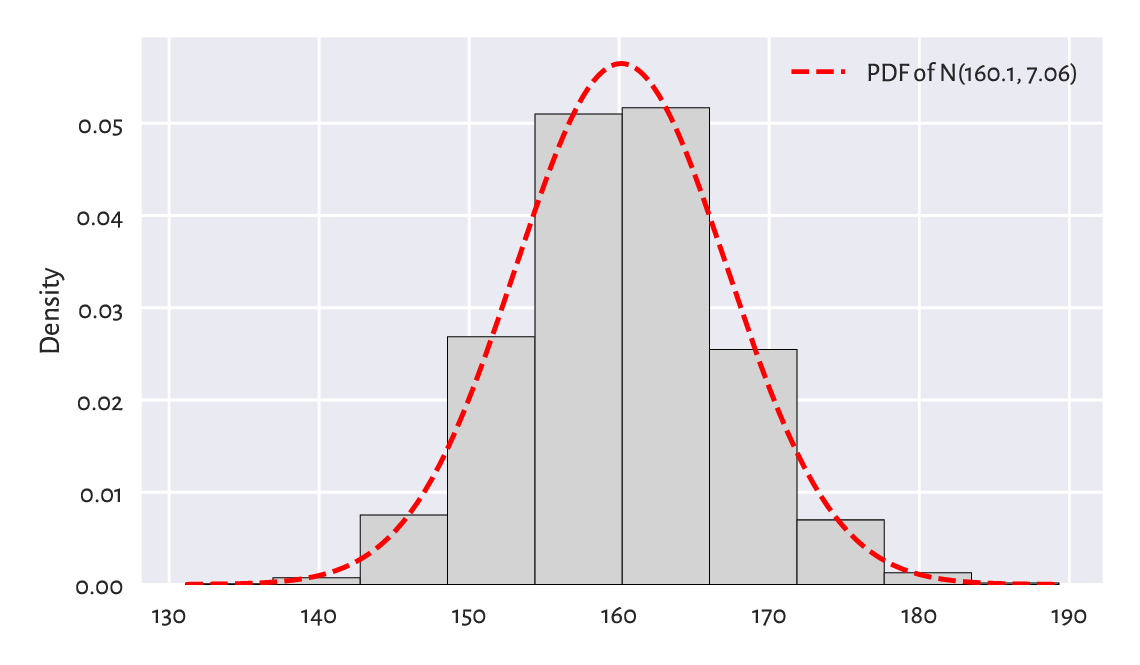Figure 6.2 A histogram and the probability density function of the fitted normal distribution for the heights dataset.#

At first glance, this is a genuinely nice match. Before proceeding with an overview of the ways to assess the goodness-of-fit more rigorously, we should praise the potential benefits of having an idealised model of our dataset at our disposal.

### 6.1.2. Data models are useful#

If (provided that, assuming that, on condition that) our sample is a realisation of independent random variables following a given distribution, or a data analyst judges that such an approximation might be justified or beneficial, then we have a set of many numbers reduced to merely a few parameters.

In the above case, we might want to risk the statement that data follow the normal distribution (assumption 1) with parameters $$\mu=160.1$$ and $$\sigma=7.06$$ (assumption 2). Still, the choice of the distribution family is one thing, and the way we estimate the underlying parameters (in our case, we use $$\hat{\mu}$$ and $$\hat{\sigma}$$) is another.

This not only saves storage space and computational time, but also – based on what we can learn from a course in probability and statistics (by appropriately integrating the PDF) – we can imply facts such as for normally distributed data:

• c. 68% of (i.e., a majority) women are $$\mu\pm \sigma$$ tall (the $$1\sigma$$ rule),

• c. 95% of (i.e., most typical) women are $$\mu\pm 2\sigma$$ tall (the $$2\sigma$$ rule),

• c. 99.7% of (i.e., almost all) women are $$\mu\pm 3\sigma$$ tall (the $$3\sigma$$ rule).

Also, if we knew that the distribution of heights of men is also normal with some other parameters, we could be able to make some comparisons between the two samples. For example, we could compute the probability that a woman randomly selected from the crowd is taller than a male passerby.

Furthermore, there is a range of parametric (assuming some distribution family) statistical methods that could additionally be used if we assumed the data normality, e.g., the t-test to compare the expected values.

Exercise 6.1

How different manufacturing industries (e.g., clothing) can make use of such models? Are simplifications necessary when dealing with complexity? What are the alternatives?

Important

We are always expected to verify the assumptions of a model that we wish to apply in practice. In particular, we will soon note that incomes are not normally distributed. Therefore, we must not refer to the above $$2\sigma$$ or $$3\sigma$$ rule in their case. A cow neither barks nor can it serve as a screwdriver. Period.

## 6.2. Assessing goodness-of-fit#

### 6.2.1. Comparing cumulative distribution functions#

Bell-shaped histograms are encountered quite frequently in real-world data: e.g., measurement errors in physical experiments and standardised tests’ results (like IQ and other ability scores) tend to be distributed this way, at least approximately.

If we yearn for more precision, there is a better way of assessing the extent to which a sample deviates from a hypothesised distribution. Namely, we can measure the discrepancy between some theoretical cumulative distribution function (CDF) and the empirical one ($$\hat{F}_n$$; see Section 4.3.8).

Important

If $$f$$ is a PDF, then the corresponding theoretical CDF is defined as $$F(x) = \int_{-\infty}^x f(t)\,dt$$, i.e., the probability of the underlying random variable’s taking a value less than or equal to $$x$$.

By definition, each CDF takes values in the unit interval ($$[0, 1]$$) and is nondecreasing.

For the normal distribution family, the values of the theoretical CDF can be computed by calling scipy.stats.norm.cdf; see Figure 6.3.

x = np.linspace(np.min(heights), np.max(heights), 1001)
probs = scipy.stats.norm.cdf(x, mu, sigma)  # sample the CDF at many points
plt.plot(x, probs, "r--", label=f"CDF of N({mu:.1f}, {sigma:.2f})")
heights_sorted = np.sort(heights)
plt.plot(heights_sorted, np.arange(1, n+1)/n,
drawstyle="steps-post", label="Empirical CDF")
plt.xlabel("$x$")
plt.ylabel("Prob(height $\\leq$ x)")
plt.legend()
plt.show()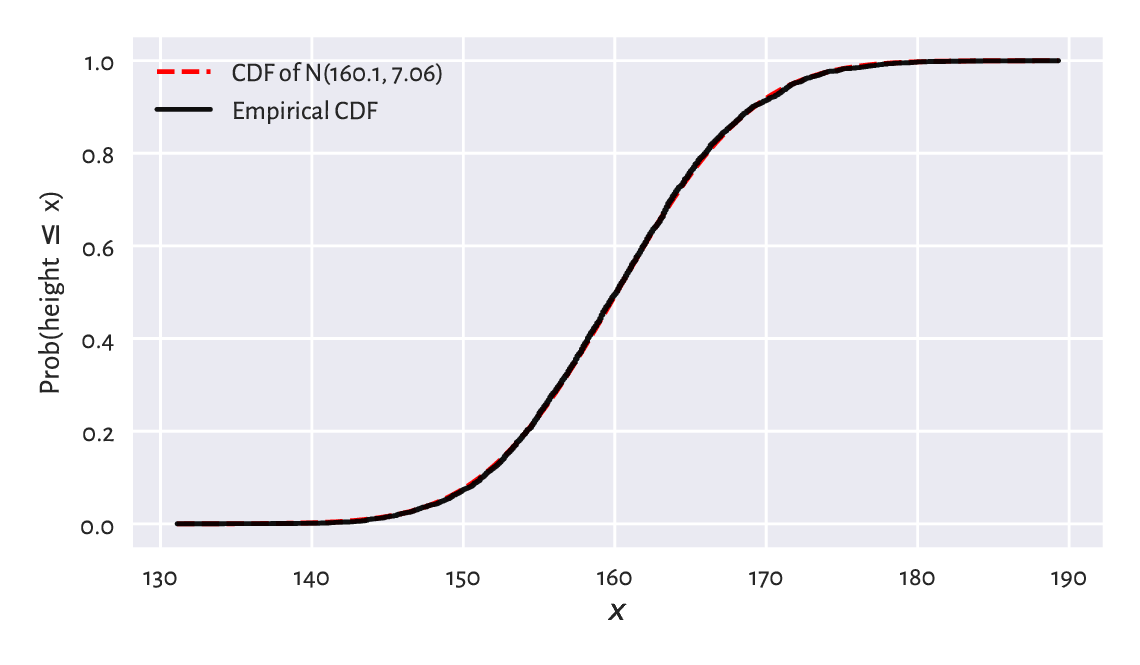Figure 6.3 The empirical CDF and the fitted normal CDF for the heights dataset: the fit is superb.#

This looks like a superb match.

Example 6.2

$$F(b)-F(a)=\int_{a}^b f(t)\,dt$$ is the probability of generating a value in the interval $$[a, b]$$.

Let us empirically verify the $$3\sigma$$ rule:

F = lambda x: scipy.stats.norm.cdf(x, mu, sigma)
F(mu+3*sigma) - F(mu-3*sigma)
## 0.9973002039367398


Indeed, almost all observations are within $$[\mu-3\sigma, \mu+3\sigma]$$, if data are normally distributed.

Note

A common way to summarise the discrepancy between the empirical and a given theoretical CDF is by computing the greatest absolute deviation:

$\hat{D}_n = \sup_{t\in\mathbb{R}} | \hat{F}_n(t) - F(t) |,$

where the supremum is a continuous version of the maximum.

It holds:

$\hat{D}_n = \max \left\{ \max_{k=1,\dots,n}\left\{ \left|\tfrac{k-1}{n} - F(x_{(k)})\right| \right\}, \max_{k=1,\dots,n}\left\{ \left|\tfrac{k}{n} - F(x_{(k)})\right| \right\} \right\},$

i.e., $$F$$ needs to be probed only at the $$n$$ points from the sorted input sample.

def compute_Dn(x, F):  # equivalent to scipy.stats.kstest(x, F)
Fx = F(np.sort(x))
n = len(x)
k = np.arange(1, n+1)  # 1, 2, ..., n
Dn1 = np.max(np.abs((k-1)/n - Fx))
Dn2 = np.max(np.abs(k/n - Fx))
return max(Dn1, Dn2)

Dn = compute_Dn(heights, F)
Dn
## 0.010470976524201148


If the difference is sufficiently small, then we can assume that a normal model describes data quite well. This is indeed the case here: we may estimate the probability of someone being as tall as any given height with an error less than about 1.05%.

### 6.2.2. Comparing quantiles#

A Q-Q plot (quantile-quantile or probability plot) is another graphical method for comparing two distributions. This time, instead of working with a cumulative distribution function $$F$$, we will be dealing with its (generalised) inverse, i.e., the quantile function $$Q$$.

Given a CDF $$F$$, the corresponding quantile function is defined for any $$p\in(0, 1)$$ as:

$Q(p) = \inf\{ x: F(x) \ge p \},$

i.e., the smallest $$x$$ such that the probability of drawing a value not greater than $$x$$ is at least $$p$$.

Important

If a CDF $$F$$ is continuous, and this is the assumption in the current chapter, then $$Q$$ is exactly its inverse, i.e., it holds $$Q(p)=F^{-1}(p)$$ for all $$p\in(0, 1)$$; compare Figure 6.4.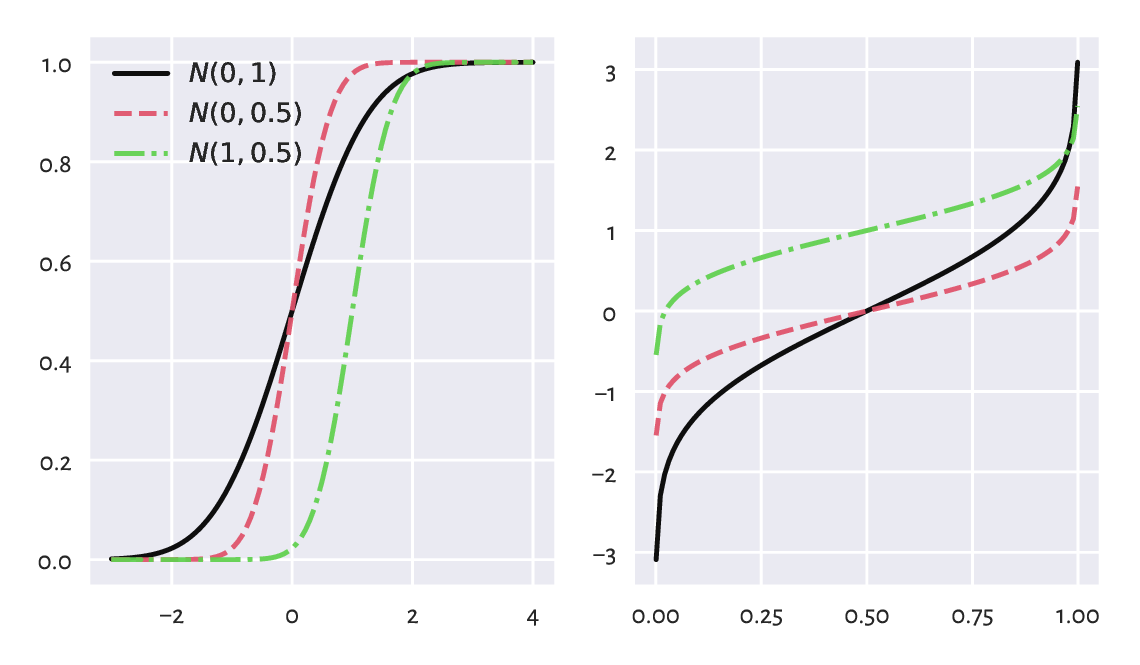Figure 6.4 The cumulative distribution functions (left) and the quantile functions (being the inverse of the CDF; right) of some normal distributions.#

The theoretical quantiles can be generated by the scipy.stats.norm.ppf function. Here, ppf stands for the percent point function which is another (yet quite esoteric) name for the above $$Q$$.

For instance, in our $$\mathrm{N}(160.1, 7.06)$$-distributed heights dataset, $$Q(0.9)$$ is the height not exceeded by 90% of the female population. In other words, only 10% of American women are taller than:

scipy.stats.norm.ppf(0.9, mu, sigma)
## 169.18820963937648


A Q-Q plot draws a version of sample quantiles as a function of the corresponding theoretical quantiles. The sample quantiles, introduced in Section 5.1.1.3, are natural estimators of the theoretical quantile function. However, we also mentioned that there are quite a few possible definitions thereof in the literature; compare .

For simplicity, instead of using numpy.quantile, we will assume that the $$\frac{i}{n+1}$$-quantile is equal to $$x_{(i)}$$, i.e., the $$i$$-th smallest value in a given sample $$(x_1,x_2,\dots,x_n)$$ and consider only $$i=1, 2, \dots, n$$.

Our simplified setting avoids the problem which arises when the 0- or 1-quantiles of the theoretical distribution, i.e., $$Q(0)$$ or $$Q(1)$$, are infinite (and this is the case for the normal distribution family).

def qq_plot(x, Q):
"""
Draws a Q-Q plot, given:
* x - a data sample (vector)
* Q - a theoretical quantile function
"""
n = len(x)
q = np.arange(1, n+1)/(n+1)    # 1/(n+1), 2/(n+2), ..., n/(n+1)
x_sorted = np.sort(x)          # sample quantiles
quantiles = Q(q)               # theoretical quantiles
plt.plot(quantiles, x_sorted, "o")
plt.axline((x_sorted[n//2], x_sorted[n//2]), slope=1,
linestyle=":", color="gray")  # identity line


Figure 6.5 depicts the Q-Q plot for our example dataset.

qq_plot(heights, lambda q: scipy.stats.norm.ppf(q, mu, sigma))
plt.xlabel(f"Quantiles of N({mu:.1f}, {sigma:.2f})")
plt.ylabel("Sample quantiles")
plt.show()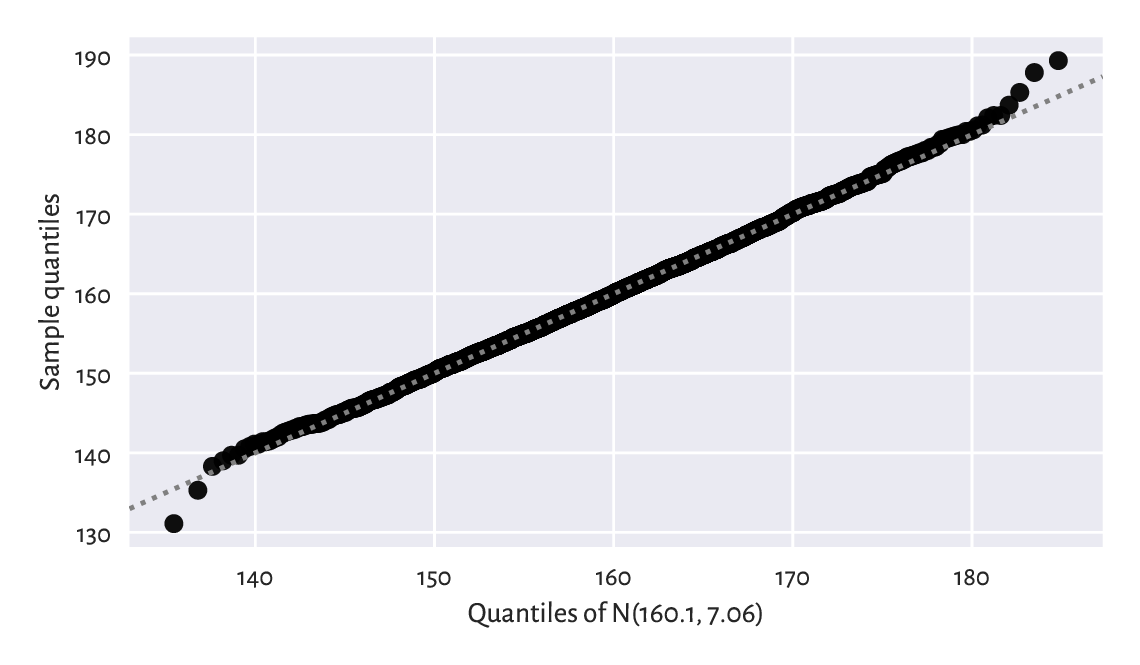Figure 6.5 The Q-Q plot for the heights dataset. It is a nice fit.#

Ideally, the points are expected to be arranged on the $$y=x$$ line (which was added for readability). This would happen if the sample quantiles matched the theoretical ones perfectly. In our case, there are small discrepancies in the tails (e.g., the smallest observation was slightly smaller than expected, and the largest one was larger than expected), although it is quite normal a behaviour for small samples and certain distribution families. Still, we can say that we observe a fine fit.

### 6.2.3. Kolmogorov–Smirnov test (*)#

To be scientific, we must yearn for some more formal method that will enable us to test the null hypothesis stating that a given empirical distribution $$\hat{F}_n$$ does not differ significantly from the theoretical continuous CDF $$F$$:

$\left\{ \begin{array}{rll} H_0: & \hat{F}_n = F & \text{(null hypothesis)}\\ H_1: & \hat{F}_n \neq F & \text{(two-sided alternative)} \\ \end{array} \right.$

The popular goodness-of-fit test by Kolmogorov and Smirnov can give us a conservative interval of the acceptable values of $$\hat{D}_n$$ (again: the largest deviation between the empirical and theoretical CDF) as a function of $$n$$ (within the framework of frequentist hypothesis testing).

Namely, if the test statistic $$\hat{D}_n$$ is smaller than some critical value $$K_n$$, then we shall deem the difference insignificant. This is to take into account the fact that reality might deviate from the ideal. Section 6.4.4 mentions that even for samples that truly come from a hypothesised distribution, there is some inherent variability. We need to be somewhat tolerant.

Any authoritative textbook in statistics will tell us (and prove) that, under the assumption that $$\hat{F}_n$$ is the ECDF of a sample of $$n$$ independent variables really generated from a continuous CDF $$F$$, the random variable $$\hat{D}_n = \sup_{t\in\mathbb{R}} | \hat{F}_n(t) - F(t) |$$ follows the Kolmogorov distribution with parameter $$n$$ (available via scipy.stats.kstwo).

In other words, if we generate many samples of length $$n$$ from $$F$$, and compute $$\hat{D}_n$$s for each of them, we expect it to be distributed like in Figure 6.6.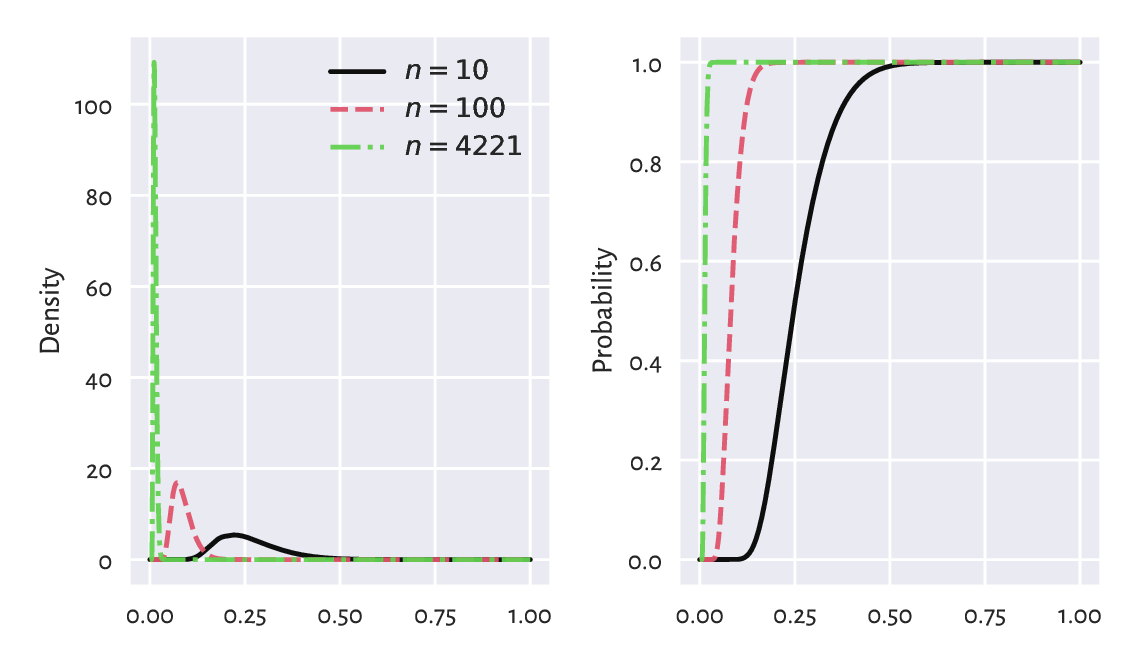Figure 6.6 Densities (left) and cumulative distribution functions (right) of some Kolmogorov distributions. The greater the sample size, the smaller the acceptable deviations between the theoretical and empirical CDFs.#

The choice $$K_n$$ involves a trade-off between our desire to:

• accept the null hypothesis when it is true (data really come from $$F$$), and

• reject it when it is false (data follow some other distribution, i.e., the difference is significant enough).

These two needs are, unfortunately, mutually exclusive.

In practice, we assume some fixed upper bound (significance level) for making the former kind of mistake, which we call the type-I error. A nicely conservative (in a good way) value that we suggest employing is $$\alpha=0.001=0.1\%$$, i.e., only 1 out of 1000 samples that really come from $$F$$ will be rejected as not coming from $$F$$.

Such a $$K_n$$ may be determined by considering the inverse of the CDF of the Kolmogorov distribution, $$\Xi_n$$. Namely, $$K_n=\Xi_n^{-1}(1-\alpha)$$:

alpha = 0.001  # significance level
scipy.stats.kstwo.ppf(1-alpha, n)
## 0.029964456376393188


In our case $$\hat{D}_n < K_n$$ because $$0.01047 < 0.02996$$. We conclude that our empirical (heights) distribution does not differ significantly (at significance level $$0.1\%$$) from the assumed one, i.e., $$\mathrm{N}(160.1, 7.06)$$. In other words, we do not have enough evidence against the statement that data are normally distributed. It is the presumption of innocence: they are normal enough.

We will go back to this discussion in Section 6.4.4 and Section 12.2.6.

## 6.3. Other noteworthy distributions#

### 6.3.1. Log-normal distribution#

We say that a sample is log-normally distributed, if its logarithm is normally distributed. Such a behaviour is frequently observed in biology and medicine (size of living tissue), social sciences (number of sexual partners), or technology (file sizes). Also, recall that Figure 6.7 reveals that this is the case for the most of the UK taxpayers’ incomes.

income = np.genfromtxt("https://raw.githubusercontent.com/gagolews/" +
"teaching-data/master/marek/uk_income_simulated_2020.txt")
plt.hist(np.log(income), bins=30, color="lightgray", edgecolor="black")
plt.ylabel("Count")
plt.show()


Let us thus proceed with the fitting of a log-normal model, L$$\mathrm{N}(\mu, \sigma)$$. The fitting process is similar to the normal case, but this time we determine the mean and standard deviation based on the logarithms of data:

lmu = np.mean(np.log(income))
lsigma = np.std(np.log(income), ddof=1)
lmu, lsigma
## (10.314409794364623, 0.5816585197803816)


We need to take note of the fact that scipy.stats.lognorm encodes the distribution via the parameter $$s$$ equal to $$\sigma$$ and scale equal to $$e^\mu$$. Computing the PDF at different points must done as follows:

x  = np.linspace(np.min(income), np.max(income), 101)
fx = scipy.stats.lognorm.pdf(x, s=lsigma, scale=np.exp(lmu))


Figure 6.8 depicts the fitted probability density function together with the histograms on the log- and original scale.

plt.subplot(1, 2, 1)
logbins = np.geomspace(np.min(income), np.max(income), 31)
plt.hist(income, bins=logbins, density=True,
color="lightgray", edgecolor="black")
plt.plot(x, fx, "r--")
plt.xscale("log")  # log-scale on the x-axis
plt.ylabel("Density")

plt.subplot(1, 2, 2)
plt.hist(income, bins=30, density=True, color="lightgray", edgecolor="black")
plt.plot(x, fx, "r--", label=f"PDF of LN({lmu:.1f}, {lsigma:.2f})")
plt.ylabel("Density")
plt.legend()

plt.show()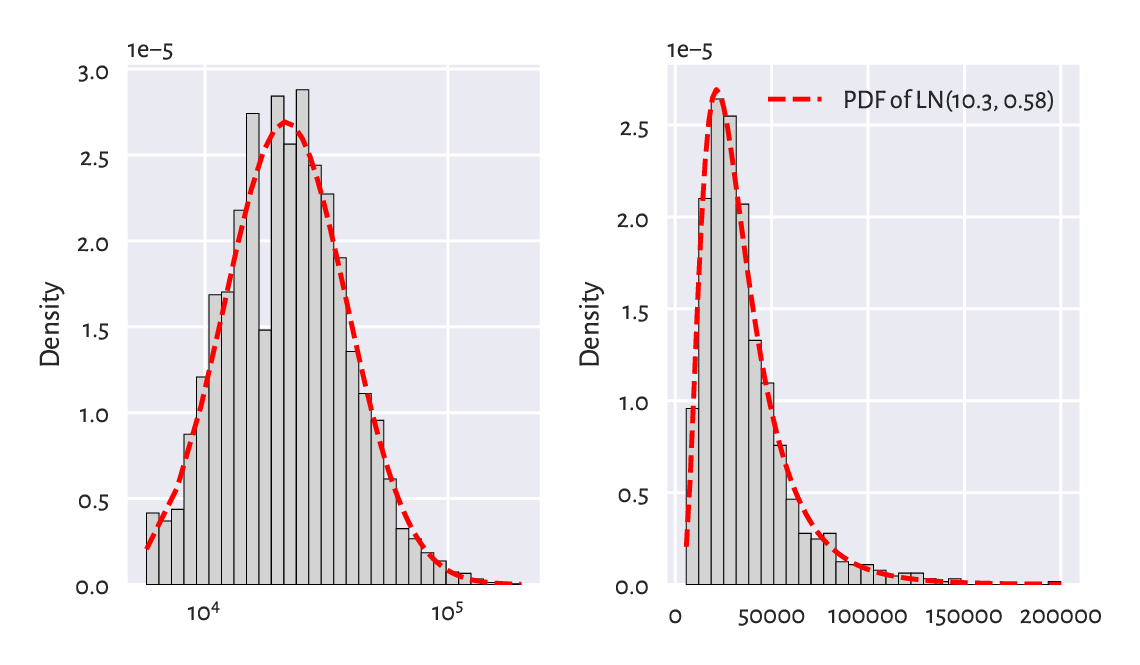Figure 6.8 A histogram and the probability density function of the fitted log-normal distribution for the income dataset, on log- (left) and original (right) scale.#

Overall, this fit is not too bad. Nonetheless, we are only dealing with a sample of 1000 households; the original UK Office of National Statistics data could tell us more about the quality of this model in general, but it is beyond the scope of our simple exercise.

Furthermore, Figure 6.9 gives the quantile-quantile plot on a double logarithmic scale for the above log-normal model. Additionally, we (empirically) verify the hypothesis of normality (using a “normal” normal distribution, not its “log” version).

plt.subplot(1, 2, 1)
qq_plot(  # see above for the definition
income,
lambda q: scipy.stats.lognorm.ppf(q, s=lsigma, scale=np.exp(lmu))
)
plt.xlabel(f"Quantiles of LN({lmu:.1f}, {lsigma:.2f})")
plt.ylabel("Sample quantiles")
plt.xscale("log")
plt.yscale("log")

plt.subplot(1, 2, 2)
mu = np.mean(income)
sigma = np.std(income, ddof=1)
qq_plot(income, lambda q: scipy.stats.norm.ppf(q, mu, sigma))
plt.xlabel(f"Quantiles of N({mu:.1f}, {sigma:.2f})")

plt.show()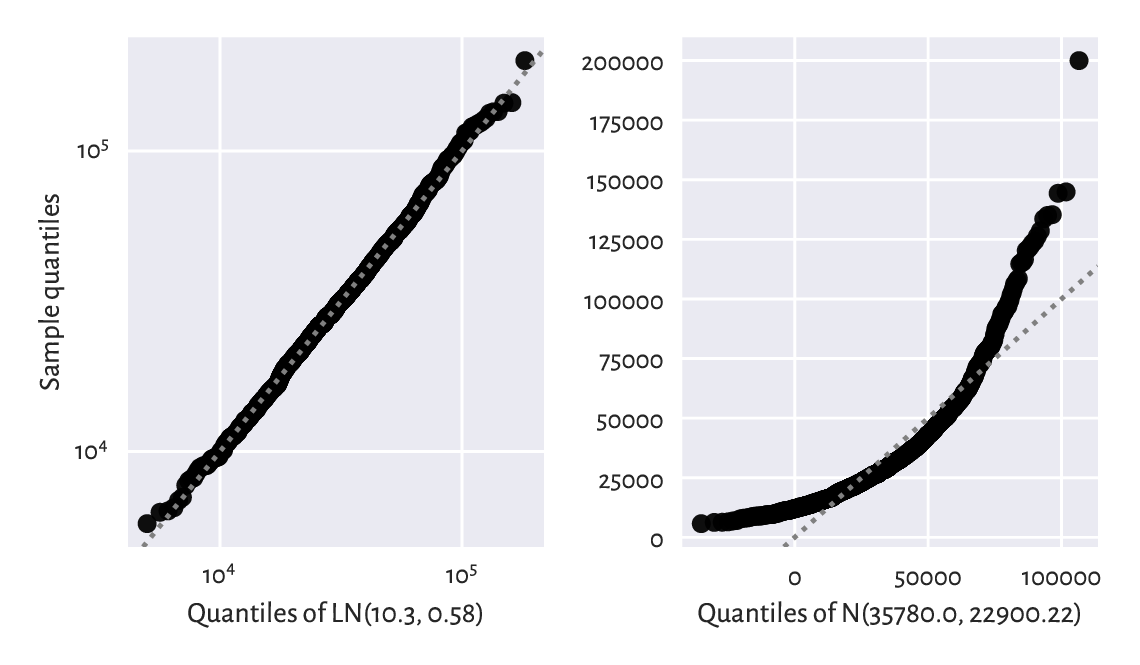Figure 6.9 The Q-Q plots for the income dataset vs a fitted log-normal (good fit; left) and normal (bad fit; right) distribution.#
Exercise 6.3

Graphically compare the empirical CDF for income and the theoretical CDF of $$\mathrm{LN}(10.3, 0.58)$$.

Exercise 6.4

(*) Perform the Kolmogorov–Smirnov goodness-of-fit test as in Section 6.2.3, to verify that the hypothesis of log-normality is not rejected at the $$\alpha=0.001$$ significance level. At the same time, the income distribution significantly differs from a normal one.

The hypothesis that our data follow a normal distribution is most likely false. On the other hand, the log-normal model, might be quite adequate. It again reduced the whole dataset to merely two numbers, $$\mu$$ and , based on which (and probability theory), we may deduce that:

• the expected average (mean) income is $$e^{\mu + \sigma^2/2}$$,

• median is $$e^\mu$$,

• most probable one (mode) in $$e^{\mu-\sigma^2}$$,

etc.

Note

Recall again that for skewed distributions such as this one, reporting the mean might be misleading. This is why most people get angry when they read the news about the prospering economy (“yeah, we’d like to see that kind of money in our pockets”). Hence, it is not only $$\mu$$ that matters, it is also $$\sigma$$ that quantifies the discrepancy between the rich and the poor (too much inequality is bad, but also too much uniformity is to be avoided).

For a normal distribution, the situation is vastly different. The mean, the median, and the most probable outcomes tend to be the same: the distribution is symmetric around $$\mu$$.

Exercise 6.5

What is the fraction of people with earnings below the mean in our $$\mathrm{LN}(10.3, 0.58)$$ model? Hint: use scipy.stats.lognorm.cdf to get the answer.

### 6.3.2. Pareto distribution#

Consider again the dataset on the populations of the US cities in the 2000 US Census:

cities = np.genfromtxt("https://raw.githubusercontent.com/gagolews/" +
"teaching-data/master/other/us_cities_2000.txt")
len(cities), sum(cities)  # number of cities, total population
## (19447, 175062893.0)


Figure 6.10 gives the histogram of the city sizes with the populations on the log-scale. It kind of looks like a log-normal distribution again, which the readers can inspect themselves when they are feeling playful.

logbins = np.geomspace(np.min(cities), np.max(cities), 21)
plt.hist(cities, bins=logbins, color="lightgray", edgecolor="black")
plt.xscale("log")
plt.ylabel("Count")
plt.show()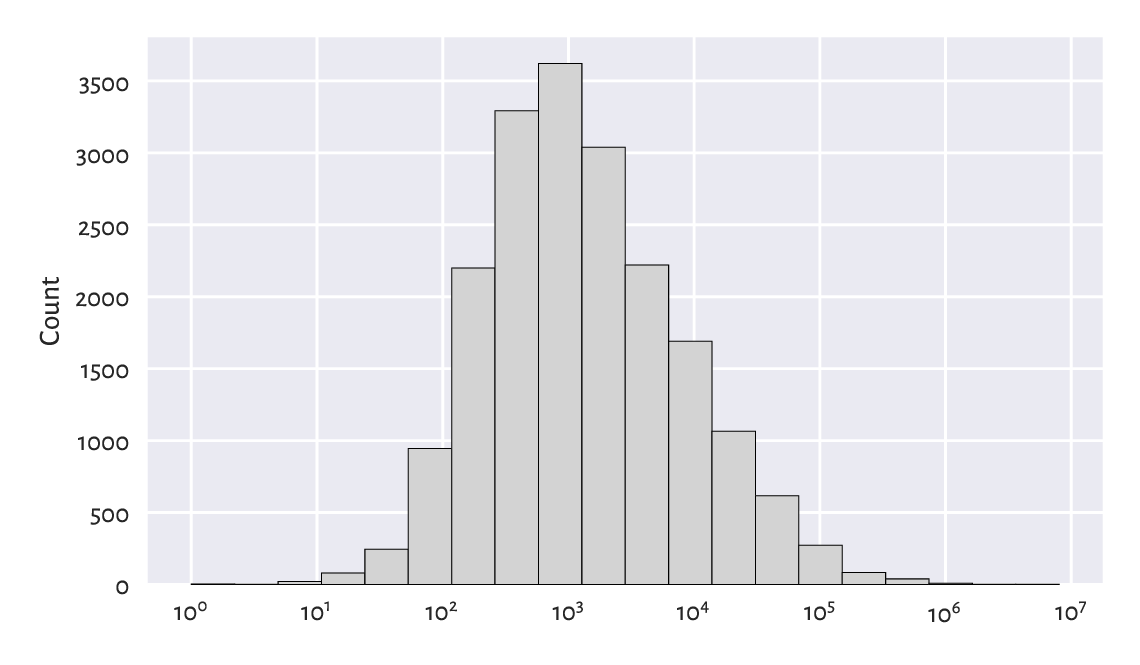Figure 6.10 A histogram of the unabridged cities dataset. Note the log-scale on the x-axis.#

This time, however, we will be interested in not what is typical, but what is in some sense anomalous or extreme. Let us look again at the truncated version of the city size distribution by considering the cities with 10 000 or more inhabitants (i.e., we will only study the right tail of the original data, just like in Section 4.3.7).

s = 10_000
large_cities = cities[cities >= s]
len(large_cities), sum(large_cities)  # number of cities, total population
## (2696, 146199374.0)


Plotting the above on a double logarithmic scale can be performed by calling plt.yscale("log"), which is left as an exercise. Anyway, doing so will lead to a picture similar to Figure 6.11 below. This reveals something remarkable. The bar tops on the double log-scale are arranged more or less in a straight line.

There are many datasets that exhibit this behaviour. We say that they follow a power law (power in the arithmetic sense, not social one); see [14, 68] for discussion.

Let us introduce the Pareto distribution family which has a prototypical power law-like density. It is identified by two parameters:

• the (what scipy calls it) scale parameter $$s>0$$ is equal to the shift from $$0$$,

• the shape parameter, $$\alpha>0$$, controls the slope of the said line on the double log-scale.

The probability density function of $$\mathrm{P}(\alpha, s)$$ is given for $$x\ge s$$ by:

$f(x) = \frac{\alpha s^\alpha}{x^{\alpha+1}},$

and $$f(x)=0$$ otherwise.

$$s$$ is usually taken as the sample minimum (i.e., 10 000 in our case). $$\alpha$$ can be estimated through the reciprocal of the mean of the scaled logarithms of our observations:

alpha = 1/np.mean(np.log(large_cities/s))
alpha
## 0.9496171695997675


Figure 6.11 allows us to compare the theoretical density and an empirical histogram on the log-scale.

logbins = np.geomspace(s, np.max(large_cities), 21)  # bin boundaries
plt.hist(large_cities, bins=logbins, density=True,
color="lightgray", edgecolor="black")
plt.plot(logbins, scipy.stats.pareto.pdf(logbins, alpha, scale=s),
"r--", label=f"PDF of P({alpha:.3f}, {s})")
plt.xscale("log")
plt.yscale("log")
plt.ylabel("Density")
plt.legend()
plt.show()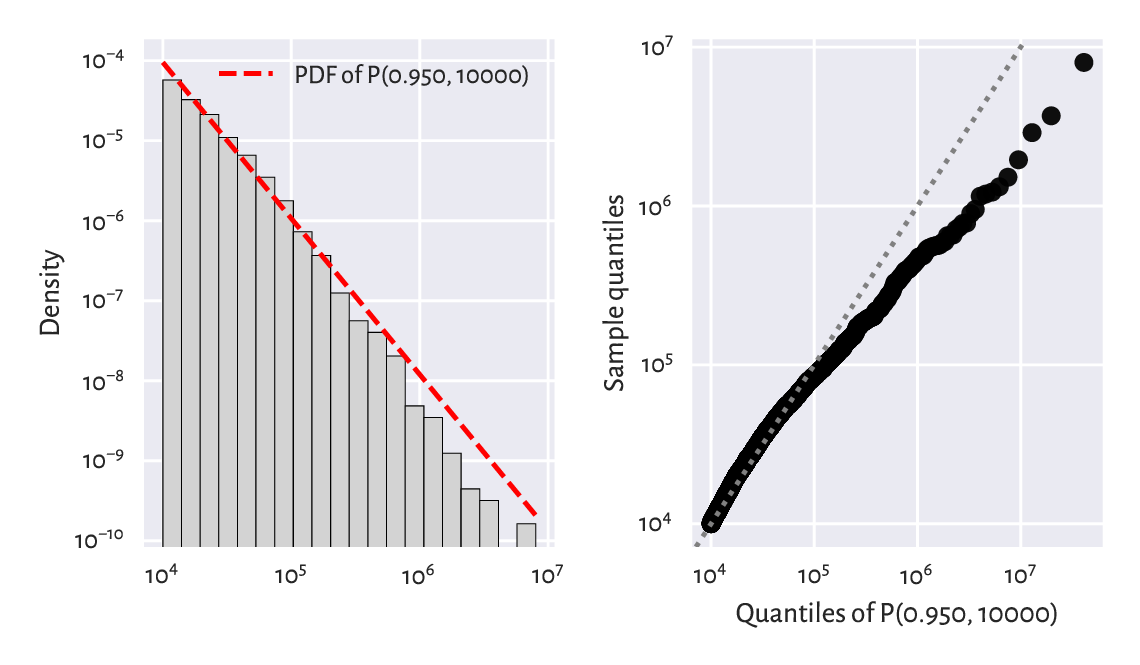Figure 6.11 A histogram of the large_cities dataset and the fitted density on a double log-scale.#

Figure 6.12 gives the corresponding Q-Q plot on a double logarithmic scale.

qq_plot(  # defined above
large_cities,
lambda q: scipy.stats.pareto.ppf(q, alpha, scale=s)
)
plt.xlabel(f"Quantiles of P({alpha:.3f}, {s})")
plt.ylabel("Sample quantiles")
plt.xscale("log")
plt.yscale("log")
plt.show()Figure 6.12 The Q-Q plot for the large_cites dataset vs the fitted Paretian model.#

We see that the populations of the largest cities are overestimated. The model could be better, but the cities are still growing, right?

Example 6.6

(*) It might also be interesting to see how well we can predict the probability of a randomly selected city being at least a given size. Let us denote by $$S(x)=1-F(x)$$ the complementary cumulative distribution function (CCDF; sometimes referred to as the survival function), and by $$\hat{S}_n(x)=1-\hat{F}_n(x)$$ its empirical version. Figure 6.13 compares the empirical and the fitted CCDFs with probabilities on the linear- and log-scale.

x = np.geomspace(np.min(large_cities), np.max(large_cities), 1001)
probs = scipy.stats.pareto.cdf(x, alpha, scale=s)
n = len(large_cities)
for i in [1, 2]:
plt.subplot(1, 2, i)
plt.plot(x, 1-probs, "r--", label=f"CCDF of P({alpha:.3f}, {s})")
plt.plot(np.sort(large_cities), 1-np.arange(1, n+1)/n,
drawstyle="steps-post", label="Empirical CCDF")
plt.xlabel("$x$")
plt.xscale("log")
plt.yscale(["linear", "log"][i-1])
if i == 1:
plt.ylabel("Prob(city size > x)")
plt.legend()
plt.show()Figure 6.13 The empirical and theoretical complementary cumulative distribution functions for the large_cities dataset with probabilities on the linear- (left) and log-scale (right) and city sizes on the log-scale.#

In terms of the maximal absolute distance between the two functions, $$\hat{D}_n$$, from the left plot we see that the fit seems acceptable. Still, let us stress that the log-scale overemphasises the relatively minor differences in the right tail and should not be used for judging the value of $$\hat{D}_n$$.

However, that the Kolmogorov–Smirnov goodness-of-fit test rejects the hypothesis of Paretianity (at a significance level $$0.1\%$$) is left as an exercise for the reader.

### 6.3.3. Uniform distribution#

Consider the Polish Lotto lottery, where six numbered balls $$\{1,2,\dots,49\}$$ are drawn without replacement from an urn. We have a dataset that summarises the number of times each ball has been drawn in all the games in the period 1957–2016.

lotto = np.genfromtxt("https://raw.githubusercontent.com/gagolews/" +
"teaching-data/master/marek/lotto_table.txt")
lotto
## array([720., 720., 714., 752., 719., 753., 701., 692., 716., 694., 716.,
##        668., 749., 713., 723., 693., 777., 747., 728., 734., 762., 729.,
##        695., 761., 735., 719., 754., 741., 750., 701., 744., 729., 716.,
##        768., 715., 735., 725., 741., 697., 713., 711., 744., 652., 683.,
##        744., 714., 674., 654., 681.])


Each event seems to occur more or less with the same probability. Of course, the numbers on the balls are integer, but in our idealised scenario, we may try modelling this dataset using a continuous uniform distribution, which yields arbitrary real numbers on a given interval $$(a, b)$$, i.e., between some $$a$$ and $$b$$. We denote such a distribution by $$\mathrm{U}(a, b)$$. It has the probability density function given for $$x\in(a, b)$$ by:

$f(x) = \frac{1}{b-a},$

and $$f(x)=0$$ otherwise.

Notice that scipy.stats.uniform uses parameters a and scale equal to $$b-a$$ instead.

In our case, it makes sense to set $$a=1$$ and $$b=50$$ and interpret an outcome like 49.1253 as representing the 49th ball (compare the notion of the floor function, $$\lfloor x\rfloor$$).

x = np.linspace(1, 50, 1001)
plt.bar(np.arange(1, 50), width=1, height=lotto/np.sum(lotto),
color="lightgray", edgecolor="black", alpha=0.8, align="edge")
plt.plot(x, scipy.stats.uniform.pdf(x, 1, scale=49), "r--",
label="PDF of U(1, 50)")
plt.ylim(0, 0.025)
plt.legend()
plt.show()


Visually, see Figure 6.14, this model makes much sense, but again, some more rigorous statistical testing would be required to determine if someone has not been tampering with the lottery results, i.e., if data does not deviate from the uniform distribution significantly.

Unfortunately, we cannot use the Kolmogorov–Smirnov test in the version defined above as data are not continuous. See, however, Section 11.4.3 for the Pearson chi-squared test that is applicable here.

Exercise 6.7

Does playing lotteries and engaging in gambling make rational sense at all, from the perspective of an individual player? Well, we see that 16 is the most frequently occurring outcome in Lotto, maybe there’s some magic in it? Also, some people sometimes became millionaires, right?

Note

In data modelling (e.g., Bayesian statistics), sometimes a uniform distribution is chosen as a placeholder for “we know nothing about a phenomenon, so let us just assume that every event is equally likely”. Nonetheless, it is quite fascinating that the real world tends to be structured after all. Emerging patterns are plentiful, most often they are far from being uniformly distributed. Even more strikingly, they are subject to quantitative analysis.

### 6.3.4. Distribution mixtures (*)#

Some datasets may fail to fit through simple models such as the ones described above. It may sometimes be due to their non-random behaviour: statistics gives just one means to create data idealisations, we also have partial differential equations, approximation theory, graphs and complex networks, agent-based modelling, and so forth, which might be worth giving a study (and then try).

Another reason may be that what we observe is, in fact, a mixture (creative combination) of simpler processes.

The dataset representing the December 2021 hourly averages pedestrian counts near the Southern Cross Station in Melbourne is a representative instance of such a scenario; compare Figure 4.5.

peds = np.genfromtxt("https://raw.githubusercontent.com/gagolews/" +
"teaching-data/master/marek/southern_cross_station_peds_2019_dec.txt")


It might not be a bad idea to try to fit a probabilistic (convex) combination of three normal distributions $$f_1$$, $$f_2$$, $$f_3$$, corresponding to the morning, lunchtime, and evening pedestrian count peaks. This yields the PDF:

$f(x) = w_1 f_1(x) + w_2 f_2(x) + w_3 f_3(x),$

for some coefficients $$w_1,w_2,w_3\ge 0$$ such that $$w_1+w_2+w_3=1$$.

Figure 6.15 depicts a mixture of $$\mathrm{N}(8, 1)$$, $$\mathrm{N}(12, 1)$$, and $$\mathrm{N}(17, 2)$$ with the corresponding weights of $$0.35$$, $$0.1$$, and $$0.55$$. This dataset is quite coarse-grained (we only have 24 bar heights at our disposal). Consequently, the estimated coefficients should be taken with a pinch of chilli pepper.

plt.bar(np.arange(24), width=1, height=peds/np.sum(peds),
color="lightgray", edgecolor="black", alpha=0.8)
x = np.arange(0, 25, 0.1)
p1 = scipy.stats.norm.pdf(x, 8, 1)
p2 = scipy.stats.norm.pdf(x, 12, 1)
p3 = scipy.stats.norm.pdf(x, 17, 2)
p = 0.35*p1 + 0.1*p2 + 0.55*p3  # weighted combination of 3 densities
plt.plot(x, p, "r--", label="PDF of a normal mixture")
plt.legend()
plt.show()Figure 6.15 A histogram of the peds dataset and a guesstimated mixture of three normal distributions.#

Important

It will frequently be the case in data wrangling that more complex entities (models, methods) will be arising as combinations of simpler (primitive) components. This is why we ought to spend a great deal of time studying the fundamentals.

Note

Some data clustering techniques (in particular, the $$k$$-means algorithm that we briefly discuss later in this course) could be used to split a data sample into disjoint chunks corresponding to different mixture components.

Also, it might be the case that the mixture components can be explained by another categorical variable that divides the dataset into natural groups; compare Chapter 12.

## 6.4. Generating pseudorandom numbers#

A probability distribution is useful not only for describing a dataset. It also enables us to perform many experiments on data that we do not currently have, but we might obtain in the future, to test various scenarios and hypotheses.

To do this, we can generate a random sample of independent (not related to each other) observations.

### 6.4.1. Uniform distribution#

When most people say random, they implicitly mean uniformly distributed. For example:

np.random.rand(5)
## array([0.69646919, 0.28613933, 0.22685145, 0.55131477, 0.71946897])


gives five observations sampled independently from the uniform distribution on the unit interval, i.e., $$\mathrm{U}(0, 1)$$.

The same with scipy, but this time the support will be $$(-10, 15)$$.

scipy.stats.uniform.rvs(-10, scale=25, size=5)  # from -10 to -10+25
## array([ 0.5776615 , 14.51910496,  7.12074346,  2.02329754, -0.19706205])


Alternatively, we could do that ourselves by shifting and scaling the output of the random number generator on the unit interval using the formula numpy.random.rand(5)*25-10.

### 6.4.2. Not exactly random#

We generate numbers using a computer, which is purely deterministic. Hence, we shall refer to them as pseudorandom or random-like ones (albeit they are indistinguishable from truly random, when subject to rigorous tests for randomness).

To prove it, we can set the initial state of the generator (the seed) via some number and see what values are produced:

np.random.seed(123)  # set seed
np.random.rand(5)
## array([0.69646919, 0.28613933, 0.22685145, 0.55131477, 0.71946897])


Then, we set the seed once again via the same number and see how “random” the next values are:

np.random.seed(123)  # set seed
np.random.rand(5)
## array([0.69646919, 0.28613933, 0.22685145, 0.55131477, 0.71946897])


This enables us to perform completely reproducible numerical experiments. This feature is very welcome. Truly scientific inquiries tend to nourish identical results under the same conditions.

Note

If we do not set the seed manually, it will be initialised based on the current wall time, which is different every… time. As a result, the numbers will seem random to us.

Many Python packages that we will be using in the future, including pandas and scikit-learn, rely on numpy’s random number generator. We will become used to calling numpy.random.seed to make them predictable.

Additionally, some of them (e.g., sklearn.model_selection.train_test_split or pandas.DataFrame.sample) are equipped with the random_state argument, which behaves as if we temporarily changed the seed (for just one call to that function). For instance:

scipy.stats.uniform.rvs(size=5, random_state=123)
## array([0.69646919, 0.28613933, 0.22685145, 0.55131477, 0.71946897])


This gives the same sequence as above.

### 6.4.3. Sampling from other distributions#

Generating data from other distributions is possible too; there are many rvs methods implemented in scipy.stats. For example, here is a sample from $$\mathrm{N}(100, 16)$$:

scipy.stats.norm.rvs(100, 16, size=3, random_state=50489)
## array([113.41134015,  46.99328545, 157.1304154 ])


Pseudorandom deviates from the standard normal distribution, i.e., $$\mathrm{N}(0, 1)$$, can also be generated using numpy.random.randn. As $$\mathrm{N}(100, 16)$$ is a scaled and shifted version thereof, the above is equivalent to:

np.random.seed(50489)
np.random.randn(3)*16 + 100
## array([113.41134015,  46.99328545, 157.1304154 ])


Important

Conclusions based on simulated data are trustworthy for they cannot be manipulated. Or can they?

The pseudorandom number generator’s seed used above, 50489, is quite suspicious. It might suggest that someone wanted to prove some point (in this case, the violation of the $$3\sigma$$ rule).

This is why we recommend sticking to only one seed most of the time, e.g., 123, or – when performing simulations – setting consecutive seeds for each iteration: 1, 2, ….

Exercise 6.8

Generate 1000 pseudorandom numbers from the log-normal distribution and draw a histogram thereof.

Note

(*) Having a reliable pseudorandom number generator from the uniform distribution on the unit interval is crucial as sampling from other distributions usually involves transforming independent $$\mathrm{U}(0, 1)$$ variates.

For instance, realisations of random variables following any continuous cumulative distribution function $$F$$ can be constructed through the inverse transform sampling (see [37, 78]):

1. Generate a sample $$x_1,\dots,x_n$$ independently from $$\mathrm{U}(0, 1)$$.

2. Transform each $$x_i$$ by applying the quantile function, $$y_i=F^{-1}(x_i)$$.

Now $$y_1,\dots,y_n$$ follows the CDF $$F$$.

Exercise 6.9

(*) Generate 1000 pseudorandom numbers from the log-normal distribution using inverse transform sampling.

Exercise 6.10

(**) Generate 1000 pseudorandom numbers from the distribution mixture discussed in Section 6.3.4.

### 6.4.4. Natural variability#

Even a sample truly generated from a specific distribution will deviate from it, sometimes considerably. Such effects will be especially visible for small sample sizes, but they usually disappear when the availability of data increases.

For example, Figure 6.16 depicts the histograms of nine different samples of size 100, all drawn independently from the standard normal distribution.

plt.figure(figsize=(plt.rcParams["figure.figsize"], )*2)  # width=height
for i in range(9):
plt.subplot(3, 3, i+1)
sample = scipy.stats.norm.rvs(size=100, random_state=i+1)
plt.hist(sample, density=True, bins=10,
color="lightgray", edgecolor="black")
plt.ylabel(None)
plt.xlim(-4, 4)
plt.ylim(0, 0.6)
plt.legend()
plt.show()


There is some ruggedness in the bar sizes that a naïve observer might try to interpret as something meaningful. A competent data scientist must train their eye to ignore such impurities. In this case, they are only due to random effects. Nevertheless, we must always be ready to detect cases which are worth attention.

Exercise 6.11

Repeat the above experiment for samples of sizes 10, 1 000, and 10 000.

Example 6.12

(*) Using a simple Monte Carlo simulation, we can verify (approximately) that the Kolmogorov–Smirnov goodness-of-fit test introduced in Section 6.2.3 has been calibrated properly, i.e., that for samples that really follow the assumed distribution, the null hypothesis is rejected only in roughly 0.1% of the cases.

Let us say we are interested in the null hypothesis referencing the standard normal distribution, $$\mathrm{N}(0, 1)$$, and sample size $$n=100$$. We need to generate many (we assume 10 000 below) such samples for each of which we compute and store the maximal absolute deviation from the theoretical CDF, i.e., $$\hat{D}_n$$.

n = 100
distrib = scipy.stats.norm(0, 1)  # assumed distribution: N(0, 1)
Dns = []
for i in range(10000):  # increase this for better precision
x = distrib.rvs(size=n, random_state=i+1)  # really follows distrib
Dns.append(compute_Dn(x, distrib.cdf))
Dns = np.array(Dns)


Now let us compute the proportion of cases which lead to $$\hat{D}_n$$ greater than the critical value $$K_n$$:

len(Dns[Dns >= scipy.stats.kstwo.ppf(1-0.001, n)]) / len(Dns)
## 0.0016


In theory, this should be equal to 0.001. But our values are necessarily approximate because we rely on randomness. Increasing the number of trials from 10 000 to, say, 1 000 000 will make the above estimate more precise.

It is also worth checking out that the density histogram of Dns resembles the Kolmogorov distribution that we can compute via scipy.stats.kstwo.pdf.

Exercise 6.13

(*) It might also be interesting to check out the test’s power, i.e., the probability that when the null hypothesis is false, it will actually be rejected. Modify the above code in such a way that x in the for loop is not generated from $$\mathrm{N}(0, 1)$$, but $$\mathrm{N}(0.1, 1)$$, $$\mathrm{N}(0.2, 1)$$, etc., and check the proportion of cases where we deem the sample distribution different from $$\mathrm{N}(0, 1)$$. Small differences in the location parameter $$\mu$$ are usually ignored, and this improves with sample size $$n$$.

### 6.4.5. Adding jitter (white noise)#

We mentioned that measurements might be subject to observational error. Rounding can also occur as early as the data collection phase. In particular, our heights dataset is precise up to 1 fractional digit. However, in statistics, when we say that data follow a continuous distribution, the probability of having two identical values in a sample is 0. Therefore, some data analysis methods might assume that there are no ties in the input vector, i.e., all values are unique.

The easiest way to deal with such numerical inconveniences is to add some white noise with the expected value of 0, either uniformly or normally distributed.

For example, for heights it makes sense to add some jitter from $$\mathrm{U}[-0.05, 0.05]$$:

heights_jitter = heights + (np.random.rand(len(heights))*0.1-0.05)
heights_jitter[:6]  # preview
## array([160.21704623, 152.68870195, 161.24482407, 157.3675293 ,
##        154.61663465, 144.68964596])


Adding noise also might be performed for aesthetic reasons, e.g., when drawing scatter plots.

### 6.4.6. Independence assumption#

Let us generate nine binary digits in a random fashion:

np.random.seed(251)  # HIDDEN
np.random.choice([0, 1], 9)
## array([1, 1, 1, 1, 1, 1, 1, 1, 1])


We can consider ourselves very lucky; all numbers are the same. So, the next number must be a “zero”, finally, right?

np.random.choice([0, 1], 1)
## array()


Wrong. The numbers we generate are independent of each other. There is no history. In the above model of randomness (Bernoulli trials; two possible outcomes with the same probability), there is a 50% chance of obtaining a “one” regardless of how many “ones” were observed previously.

We should not seek patterns where there are none. Our brain forms expectations about the world, which are difficult to overcome. But the reality could not care less about what we consider it to be.

For an excellent general introductory course on probability and statistics, see [38, 40] and also . More advanced students are likely to enjoy other classics such as [4, 7, 17, 26]. To go beyond the fundamentals, check out . Topics in random number generation are covered in [37, 56, 78].

For a more detailed introduction to exploratory data analysis, see the classical books by Tukey [88, 89] and Tufte .

We took the logarithm of the log-normally distributed incomes and obtained a normally distributed sample. In statistical practice, it is not rare to apply different non-linear transforms of the input vectors at the data preprocessing stage (see, e.g., Section 9.2.6). In particular, the Box–Cox (power) transform  is of the form $$x\mapsto \frac{x^\lambda-1}{\lambda}$$ for some $$\lambda$$. Interestingly, in the limit as $$\lambda\to 0$$, this formula yields $$x\mapsto \log x$$ which is exactly what we were applying in this chapter.

[14, 68] give a nice overview of the power-law-like behaviour of some “rich” or otherwise extreme datasets. It is worth noting that the logarithm of a Paretian sample divided by the minimum follows an exponential distribution (which we discuss in Chapter 16). For a comprehensive catalogue of statistical distributions, their properties, and relationships between them, see .

## 6.6. Exercises#

Exercise 6.14

Why is the notion of the mean income confusing the general public?

Exercise 6.15

When manually setting the seed of a random number generator makes sense?

Exercise 6.16

Given a log-normally distributed sample x, how can we turn it to a normally distributed one, i.e., y=f(x), with f being… what?

Exercise 6.17

What is the $$3\sigma$$ rule for normally distributed data?

Exercise 6.18

(*) How can we verify graphically if a sample follows a hypothesised theoretical distribution?

Exercise 6.19

(*) Explain the meaning of the type I error, significance level, and a test’s power.